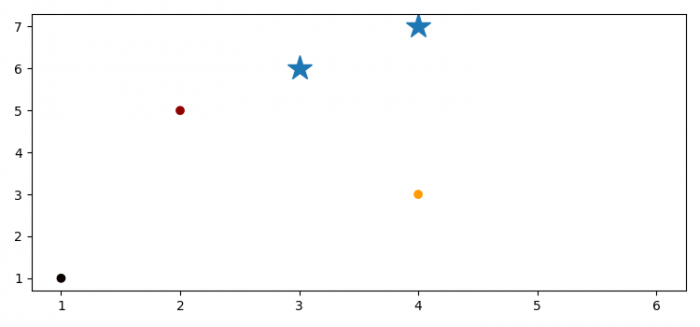# How to plot additional points on the top of a scatter plot in Matplotlib?

To plot additional points on the top of a scatter plot in matplotlib, we can take the following steps −

## Steps

• Set the figure size and adjust the padding between and around the subplots.

• Make a list of x and y data points.

• Create a scatter plot with x and y data points.

• Plot the additional points with marker='*'

• To display the figure, use show() method.

## Example

from matplotlib import pyplot as plt

# Set the figure size
plt.rcParams["figure.figsize"] = [7.50, 3.50]
plt.rcParams["figure.autolayout"] = True

# List of data points
x = [1, 2, 6, 4]
y = [1, 5, 2, 3]

# Scatter plot with x and y
plt.scatter(x, y, c=x, cmap='hot')

plt.plot([3, 4], [6, 7], marker='*', ls='none', ms=20)

# Display the plot
plt.show()

## Output

It will produce the following output −